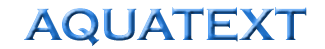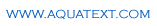The Free On-line Aquaculture DictionaryAir Lift calculation
 Maximum water flow from an airlift pump Limitations of calculation (outwith these parameters the calculations will become less accurate): Pipe diameter 1.5 - 8cm Pipe length 60 - 300 cm Q = ((0.758 x (%s)1.5 x ht0.33) + 0.01196) x d2.2 Where ; Q = Water flow rate, litres per minute ht = Total length of pipe (cm) d = diameter of pipe (cm) %s = Percent of pipe under water (measured from point of diffuser to discharge) Air requirements for optimal water flow are typically 8-9% of the water volume. Above 10%, normal bubble flow ceases and the efficiency of the airlift drops rapidly